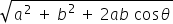science
Easy

Question

# Which disease or disorder is the result of having an extra chromosome?

## Cystic FibrosisHemophilia  ColorblindnessDown SyndromeHint:

## The correct answer is: Down Syndrome

### Down syndrome or chromosome 21 trisomy is a type of chromosomal genetic disorder. Chromosomal genetic disorder refers to change in the structure of a chromosome due to addition or deletion of chromosomal segments during cell division.In Down syndrome, the chromosome 21 will have an additional chromosome due to improper division during meiosis. The individual will posses problems in the nervous system and lack intellectual skills.

• Down syndrome is also termed as trisomy 21
• Individuals posses an extra chromosome at 21 position
• Trisomy 21 is caused due to improper separation of chromosomes during meiosis
• Cystic fibrosis is an autosomal recessive disorder
• Color blindness is an autosomal dominant disorder
• Hemophilia is a monogenic genetic disorder

science

### Which disease or disorder is caused by the inheritance of two mutated alleles?

• Cystic fibrosis results in breathing disorder of the affected individual due to increased thick mucus secretion
• The disorder is caused due to alteration in CFRT gene
• It is a type of autosomal recessive disorder, having both copies of gene altered in a cell
• Other disorders mentioned are not autosomal recessive in nature

### Which disease or disorder is caused by the inheritance of two mutated alleles?

• Cystic fibrosis results in breathing disorder of the affected individual due to increased thick mucus secretion
• The disorder is caused due to alteration in CFRT gene
• It is a type of autosomal recessive disorder, having both copies of gene altered in a cell
• Other disorders mentioned are not autosomal recessive in nature

science

science

### When does chromosomal crossover occur?science

science

science

### What is the structural and functional unit of heredity?science

science

science

### If Tan A = 1, find the value of A as directionscience

science

### If a vector has zero magnitude, then the vector is called as

Null vector is a directionless vector whose magnitude is zero and the resultant vector of two or more equal vectors that are acting opposite to each other.

### If a vector has zero magnitude, then the vector is called as

Null vector is a directionless vector whose magnitude is zero and the resultant vector of two or more equal vectors that are acting opposite to each other.

science

### Parallelogram law is used when _______

In parallelogram law of vector addition, it says that if we place two vectors so they have the same initial points(the tails are connected) and then complete the vectors into a parallelogram, then the sum of the vectors is directed diagonal that starts at the same point as the vectors.

### Parallelogram law is used when _______

In parallelogram law of vector addition, it says that if we place two vectors so they have the same initial points(the tails are connected) and then complete the vectors into a parallelogram, then the sum of the vectors is directed diagonal that starts at the same point as the vectors.science

science

science

### Two vectors started from the origin and moved to the east and north direction 10 m, 15 m, respectively, and the angle between the vectors is 30 degrees, then find the resultant of the vectors.

If two vectors A and B with magnitude a and b respectively are given and the angle between them is θ Then the magnitude of the Resultant of A and B is R =### Two vectors started from the origin and moved to the east and north direction 10 m, 15 m, respectively, and the angle between the vectors is 30 degrees, then find the resultant of the vectors.

If two vectors A and B with magnitude a and b respectively are given and the angle between them is θ Then the magnitude of the Resultant of A and B is R =#### With Turito Foundation.#### Get an Expert Advice From Turito.